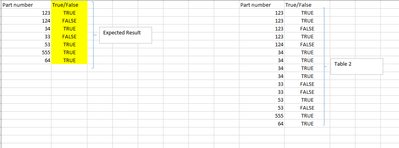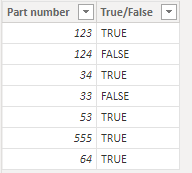cancel
Showing results for
Did you mean:Helper III

## DAX formula

Hi.

Thanks for looking at my post.

I am having 2 tables named as table 1 and table 2 but not related to each other.

What i am looking for DAX formula for "Table 1" column B as shown below, if any of the part number from column A in excel (which is table1  in power BI), match with column I in excel (which is table 2 in power bi) and if column J is True for any part number, then expected result should be true please.

There are some instance where column J will have both true and false for same part numbers and in that case True will be over ridden please.1 ACCEPTED SOLUTIONCommunity Support

Hi @jimpatel ,

Please try this code to create a calculated column in Table 1. When you use this code, please make sure the data type of [True/False] is text.

``````True/False =
VAR _List =
CALCULATETABLE (
VALUES ( 'Table 2'[True/False] ),
FILTER (
'Table 2',
'Table 2'[Part number] = EARLIER ( 'Table 1'[Part number] )
)
)
RETURN
IF ( "TRUE" IN _List, "TRUE", "FALSE" )``````

Result is as below.Best Regards,
Rico Zhou

If this post helps, then please consider Accept it as the solution to help the other members find it more quickly.

2 REPLIES 2Helper III

Hi

Thanks for looking at my post.

I am using below DAX code what should i do please to get information from same table and from same column please. That is i have attached an example "Modified status" which will look for material in the same column (it will be more than 2 in some cases) and if the match found then if "Status" is "Yes" for any of the matched part then "Modified status" will be yes for that part number please.

Any idea pleasE? got stuck with this for ages : (

Column1 =
IF (
ISEMPTY (
FILTER (
'Table1',
'Table1'[Material]= 'Table2'[Material 2]
&& 'Table1'[status] = "Yes"
)
),
"NO",
"YES"
)Community Support

Hi @jimpatel ,

Please try this code to create a calculated column in Table 1. When you use this code, please make sure the data type of [True/False] is text.

``````True/False =
VAR _List =
CALCULATETABLE (
VALUES ( 'Table 2'[True/False] ),
FILTER (
'Table 2',
'Table 2'[Part number] = EARLIER ( 'Table 1'[Part number] )
)
)
RETURN
IF ( "TRUE" IN _List, "TRUE", "FALSE" )``````

Result is as below.Best Regards,
Rico Zhou

If this post helps, then please consider Accept it as the solution to help the other members find it more quickly.